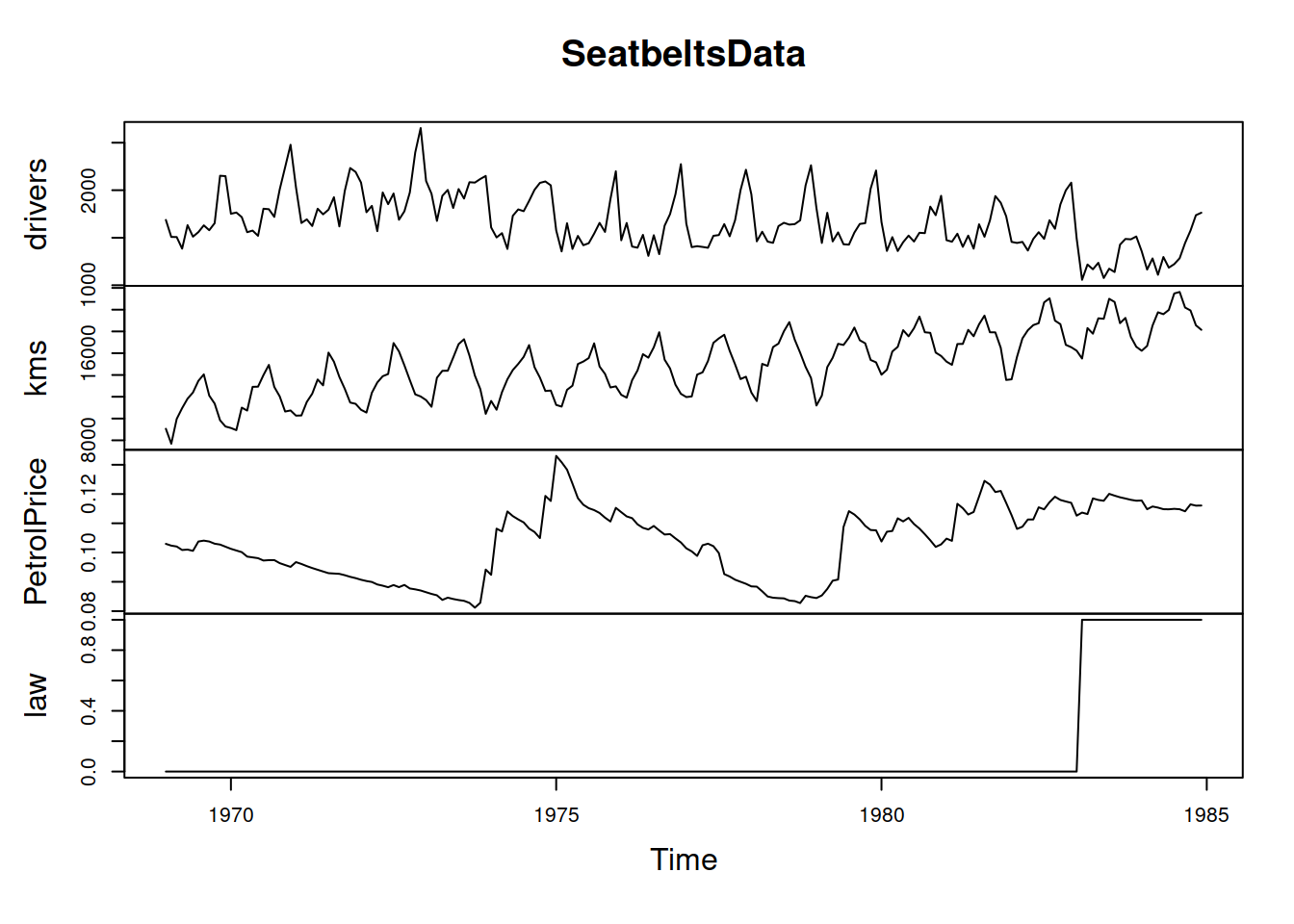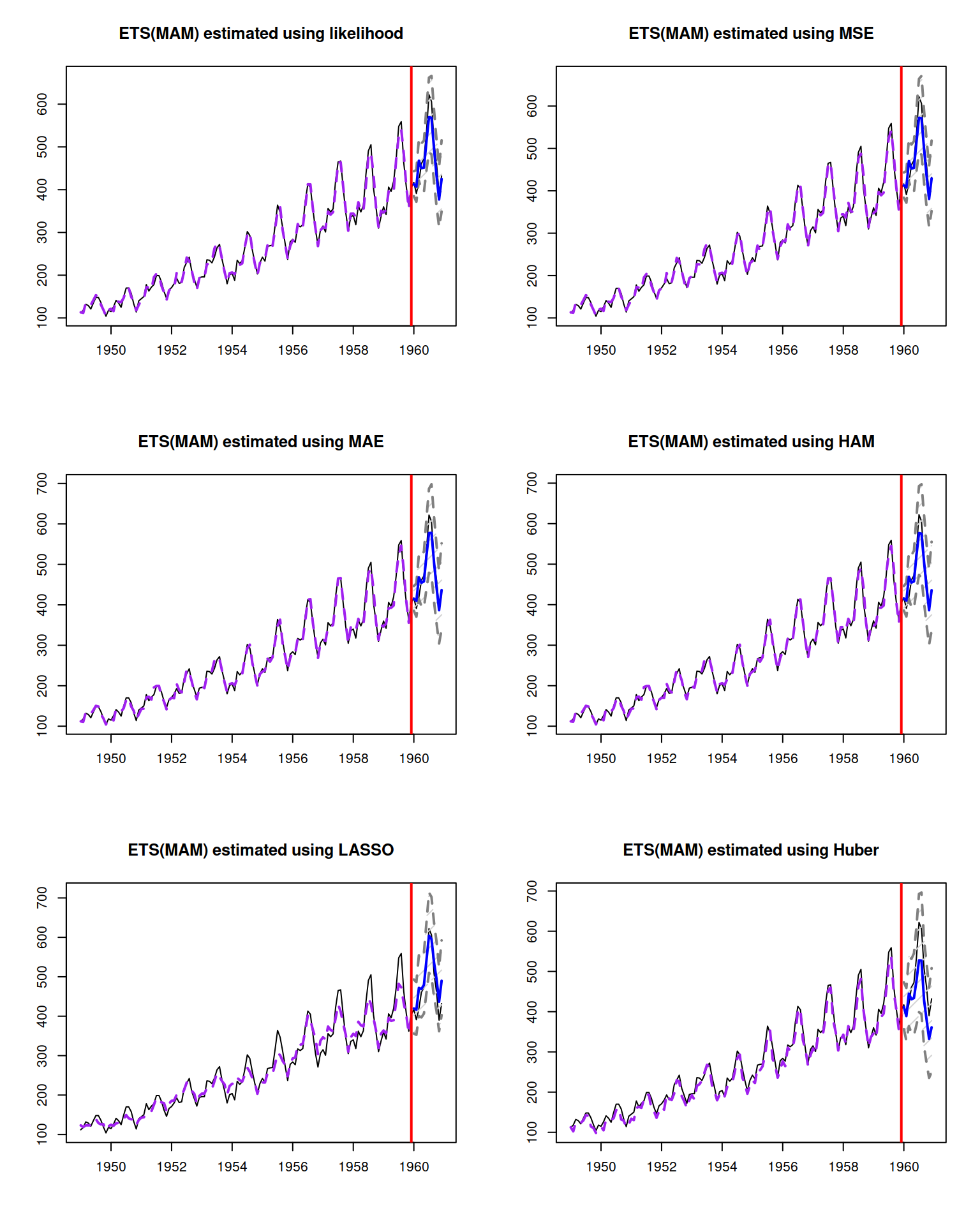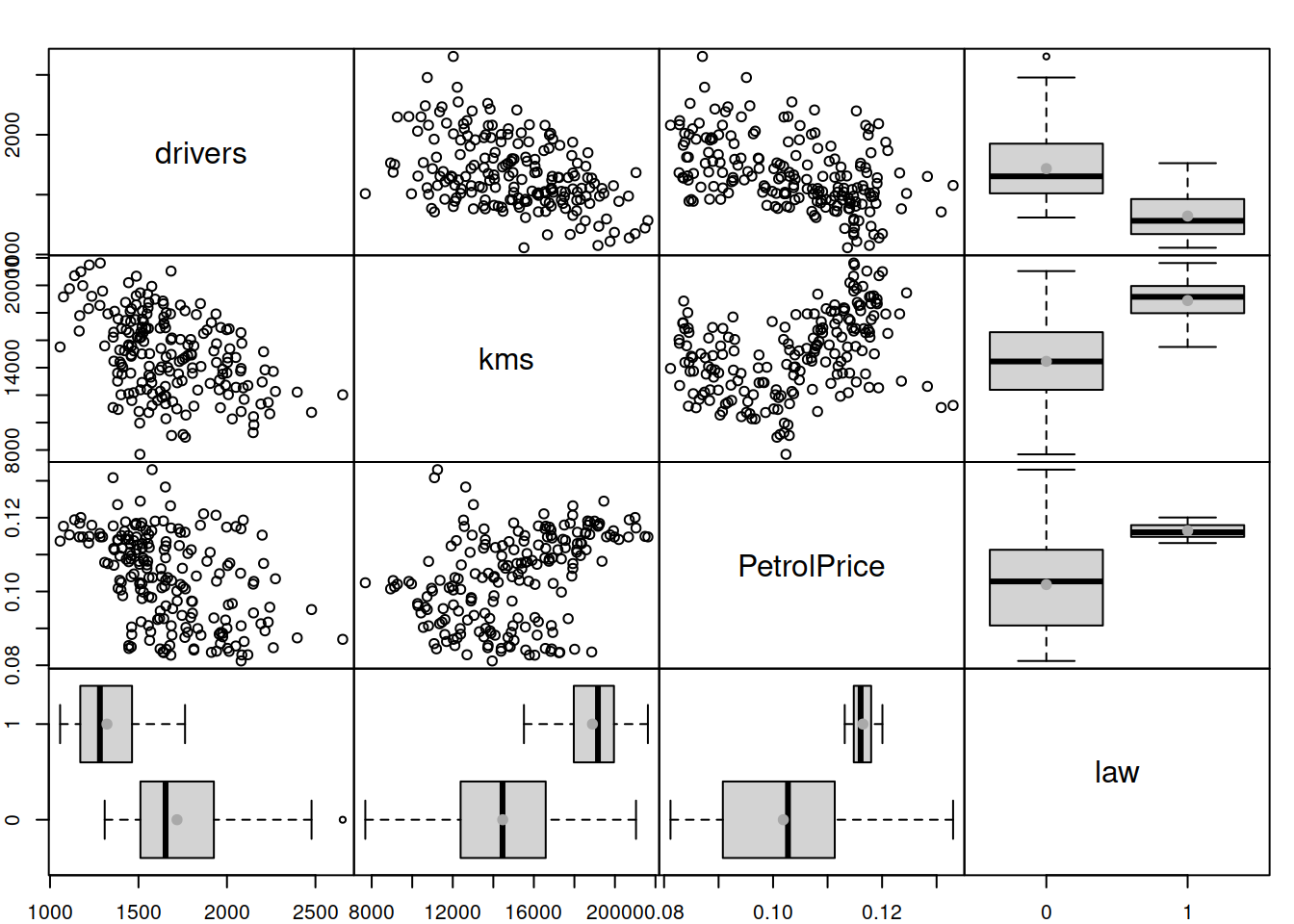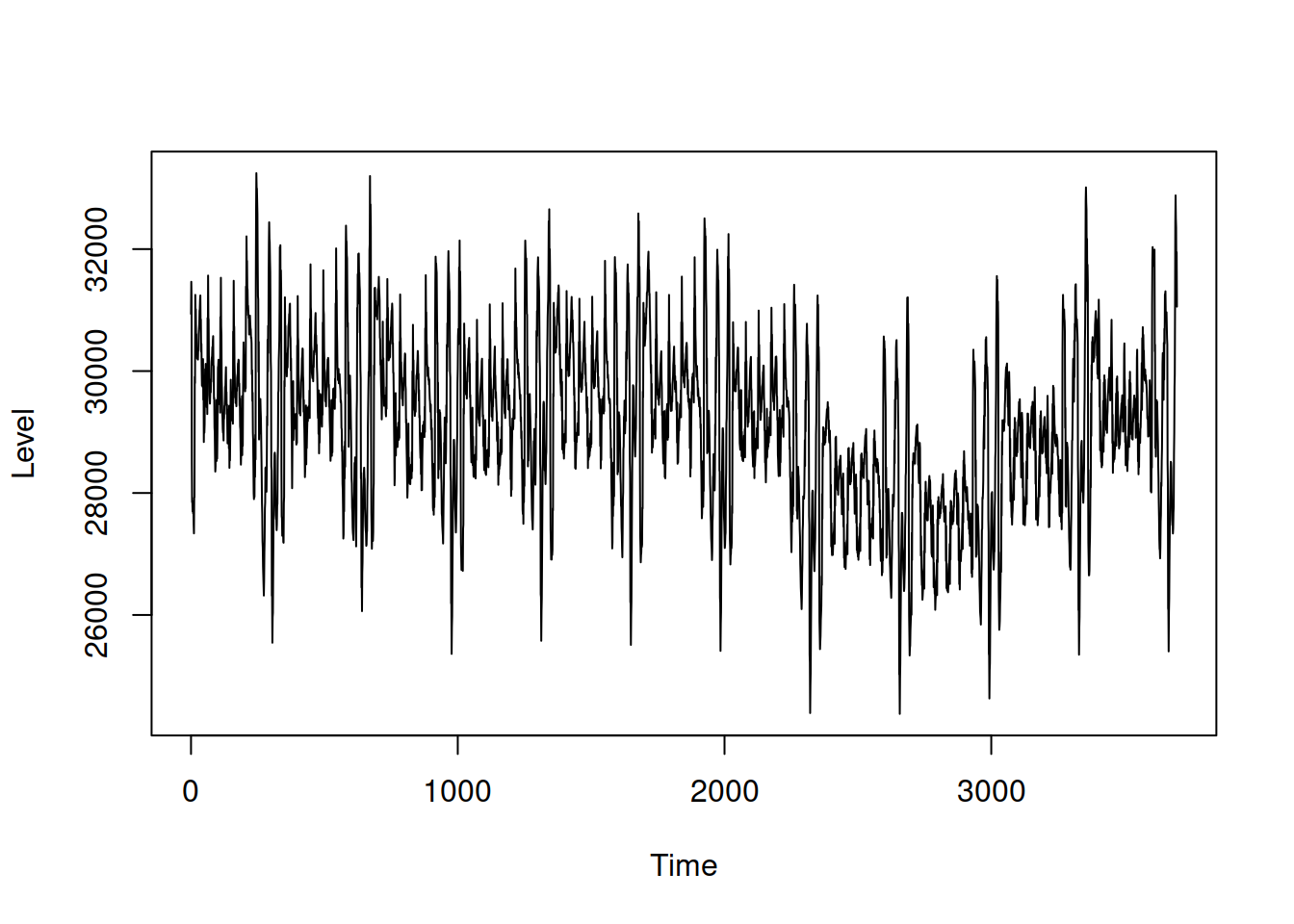This book is in Open Review. I want your feedback to make the book better for you and other readers. To add your annotation, select some text and then click the on the pop-up menu. To see the annotations of others, click the button in the upper right hand corner of the page

## 11.6 Examples of application

For this example, we will use the data of Road Casualties in Great Britain 1969–84, Seatbelts dataset in datasets package for R, which contains several variables, the description for which is provided in the documentation for the data (can be accessed via ?Seatbelts command). The variable of interest in this case is drivers, and the dataset contains more variables than needed, so we will restrict the data with drivers, kms (distance driven), PetrolPrice and law - the latter three seem to influence the number of injured / killed drivers in principle:

SeatbeltsData <- Seatbelts[,c("drivers","kms","PetrolPrice","law")]

The dynamics of these variables over time is shown on figure 11.1

plot(SeatbeltsData)Figure 11.1: The time series dynamics of variables from Seatbelts dataset.

It is apparent that the drivers variable exhibits seasonality, but does not seem to have a trend. The type of seasonality is difficult to determine, but we will assume that it is multiplicative. So a simple ETS(M,N,M) model applies to the data will produce the following (we will withhold the last 12 observations for the forecast evaluation):

adamModelETSMNM <- adam(SeatbeltsData[,"drivers"],"MNM",h=12,holdout=TRUE)
plot(forecast(adamModelETSMNM,h=12,interval="prediction"))This simple model already does a fine job in fitting and forecasting the data, although the forecast is biased and is lower than needed because of the sudden drop in the level of series, which can only be explained by the introduction of the new law in the UK in 1983, making the seatbelts compulsory for drivers. Due to the sudden drop, the smoothing parameter for the level of series is higher than needed, leading to wider intervals and less accurate forecasts, here is the output of the model:

adamModelETSMNM
## Time elapsed: 0.14 seconds
## Model estimated using adam() function: ETS(MNM)
## Distribution assumed in the model: Inverse Gaussian
## Loss function type: likelihood; Loss function value: 1126.128
## Persistence vector g:
##  alpha  gamma
## 0.4189 0.0000
##
## Sample size: 180
## Number of estimated parameters: 15
## Number of degrees of freedom: 165
## Information criteria:
##      AIC     AICc      BIC     BICc
## 2282.256 2285.182 2330.150 2337.749
##
## Forecast errors:
## ME: 117.633; MAE: 117.633; RMSE: 137.342
## sCE: 83.505%; sMAE: 6.959%; sMSE: 0.66%
## MASE: 0.682; RMSSE: 0.61; rMAE: 0.503; rRMSE: 0.541

In order to further explore the data we will produce the scatterplots and boxplots between the variables using spread() function from greybox package:

spread(SeatbeltsData)Figure 11.2: The relation between variables from Seatbelts dataset

The plot on Figure 11.2 shows that there is a negative relation between kms and drivers: the higher the distance driven, the lower the total of car drivers killed or seriously injuried. A similar relation is observed between the PetrolPrice and drivers (when the prices are high, people tend to drive less, thus causing less incidents). Interestingly, the increase of both variables causes the variance of the response variable to decrease (heteroscedasticity effect). Using multiplicative error model and including the variables in logarithms in this case might address this potential issue. Note that we do not need to take the logarithm of drivers, as we already use the model with multiplicative error. Finally, the legislation of a new law seems to have caused the decrease in the number of causalities. In order to have a better model in terms of explanatory and predictive power, we should include all three variables in the model. This is how we can make it in ADAM:

adamModelETSXMNM <- adam(SeatbeltsData,"MNM",h=12,holdout=TRUE,
formula=drivers~log(kms)+log(PetrolPrice)+law)
plot(forecast(adamModelETSXMNM,h=12,interval="prediction"))The parameter formula is not compulsory in this case and can either be substituted by formula=drivers~. or dropped completely - the function would fit the model of the first variable in the matrix from everything else. The forecast from the second model is slightly more accurate and, what is even more important, the prediction interval is narrower, because now the model takes the external information into account. Here is the summary of the second model:

adamModelETSXMNM
## Time elapsed: 0.25 seconds
## Model estimated using adam() function: ETSX(MNM)
## Distribution assumed in the model: Inverse Gaussian
## Loss function type: likelihood; Loss function value: 1115.662
## Persistence vector g (excluding xreg):
##  alpha  gamma
## 0.2098 0.0031
##
## Sample size: 180
## Number of estimated parameters: 18
## Number of degrees of freedom: 162
## Information criteria:
##      AIC     AICc      BIC     BICc
## 2267.324 2271.573 2324.798 2335.829
##
## Forecast errors:
## ME: 96.558; MAE: 98.609; RMSE: 126.2
## sCE: 68.544%; sMAE: 5.833%; sMSE: 0.557%
## MASE: 0.572; RMSSE: 0.56; rMAE: 0.422; rRMSE: 0.497

The model with explanatory variables is already more precise than the simple univariate ETS(M,N,M) (e.g. MASE on the holdout is lower), but we could try introducing the update of the parameters for the explanatory variables, just to see how it works (it might be unnecessary for this data):

adamModelETSXMNMD <- adam(SeatbeltsData,"MNM",h=12,holdout=TRUE,
plot(forecast(adamModelETSXMNMD,h=12,interval="prediction"))In this specific case, the difference between the ETSX and ETSX{D} models is infinitesimal in terms of the accuracy of final forecasts and prediction intervals. Here is the output of the model:

adamModelETSXMNMD
## Time elapsed: 0.29 seconds
## Model estimated using adam() function: ETSX(MNM){D}
## Distribution assumed in the model: Inverse Gaussian
## Loss function type: likelihood; Loss function value: 1115.373
## Persistence vector g (excluding xreg):
##  alpha  gamma
## 0.0733 0.0009
##
## Sample size: 180
## Number of estimated parameters: 21
## Number of degrees of freedom: 159
## Information criteria:
##      AIC     AICc      BIC     BICc
## 2272.745 2278.593 2339.798 2354.982
##
## Forecast errors:
## ME: 98.371; MAE: 100.787; RMSE: 126.818
## sCE: 69.831%; sMAE: 5.962%; sMSE: 0.563%
## MASE: 0.584; RMSSE: 0.563; rMAE: 0.431; rRMSE: 0.499

We can spot that the error measures of the dynamic model are a bit higher than the ones from the static one (e.g., compare MASE and RMSSE of models). In addition, the information criteria are slightly lower for the static model, so based on all of this, we should probably use the static one for the forecasting and anlytical purposes. In order to see the effect of the explanatory variables on the number of incidents with drivers, we can look at the parameters for those variables:

adamModelETSXMNM$initial$xreg
##         log.kms. log.PetrolPrice.              law
##       -0.1493740       -0.3927249       -0.2386495

Based on that, we can point out that the introduction of the law reduced on average the number of incidents by approximately 24%, while the increase of the petrol price by 1% leads on average to decrease in the number of incidents by 0.39%. Finally, the distance has a negative impact on incidents as well, reducing it on average by 0.2% for each 1% increase in the distance. All of this is the standard interpretation of parameters, which we can use based on the estimated model. We will discuss how to do analysis using ADAM in future chapters, introducing the standard errors and confidence intervals for the parameters.